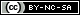# Weibull++ Examples

(Difference between revisions)
 Revision as of 22:09, 21 November 2013 (view source) (→Life Data Analysis)← Older edit Revision as of 21:11, 18 April 2014 (view source)Newer edit → Line 15: Line 15: * [http://www.reliasoft.tv/weibull/startguide/weibull_qsg_1.html View the Weibull++ example videos] * [http://www.reliasoft.tv/weibull/startguide/weibull_qsg_1.html View the Weibull++ example videos] - ==Life Data Analysis== + ==Parametric Life Data Analysis== *Simple Probability Plotting Examples: [[Probability Plotting Example|Simple Example]], [[3-Parameter_Weibull_Example|3-P Weibull Example]], [[1P_Exponential_Example|1-P Exponential]], [[Normal Distribution Probability Plotting Example|Normal]], [[Example:_Lognormal_Distribution_Probability_Plot|Lognormal]] *Simple Probability Plotting Examples: [[Probability Plotting Example|Simple Example]], [[3-Parameter_Weibull_Example|3-P Weibull Example]], [[1P_Exponential_Example|1-P Exponential]], [[Normal Distribution Probability Plotting Example|Normal]], [[Example:_Lognormal_Distribution_Probability_Plot|Lognormal]] *[[Standard_Folio_Plots|Standard Folio Plots]] *[[Standard_Folio_Plots|Standard Folio Plots]] Line 27: Line 27: :*[[Generalized_Gamma_Distribution_Example|Generalized Gamma ]] :*[[Generalized_Gamma_Distribution_Example|Generalized Gamma ]] :*[[Gumbel_Distribution_Example|Gumbel]] :*[[Gumbel_Distribution_Example|Gumbel]] - *[[Weibull++ Non-Parametric LDA Plot Example|Non-Parametric Life Data Analysis (LDA)]] *[[Bayesian-Weibull_Lognormal_Prior Example|Bayesian-Weibull Analysis]] *[[Bayesian-Weibull_Lognormal_Prior Example|Bayesian-Weibull Analysis]] *[[Mixed Weibull Example]] *[[Mixed Weibull Example]] Line 33: Line 32: :* Two Failure Modes Example. View it in '''[http://www.reliasoft.com/Weibull/examples/rc10/index.htm HTML]''' or        '''[http://www.reliasoft.tv/weibull/appexamples/weibull_app_ex_10.html Video]''' :* Two Failure Modes Example. View it in '''[http://www.reliasoft.com/Weibull/examples/rc10/index.htm HTML]''' or        '''[http://www.reliasoft.tv/weibull/appexamples/weibull_app_ex_10.html Video]''' :* [[Complex_Failure_Modes_Example|Complex Failure Modes Example]] :* [[Complex_Failure_Modes_Example|Complex Failure Modes Example]] + + ==Non-Parametric Life Data Analysis== + *[[Weibull++ Non-Parametric LDA Plot Example|Kaplan-Meier Method]] + *Simple Actuarial Method. View it in '''[http://www.reliasoft.com/Weibull/examples/rc7/index.htm HTML]''' ==Degradation Data Analysis== ==Degradation Data Analysis==

## Quick Start Guide

The Weibull++ & ALTA Quick Start Guide has been designed to help you explore many of the software's key features by working through step-by-step instructions for some practical application examples. The guide is available as a free *.PDF download and you can also watch videos posted on ReliaSoft.tv.

## Non-Parametric Life Data Analysis

• Crack Propagation Example (Point Estimation). View it in HTML or Video

## UtilitiesContent on this site is available/licensed under the "Creative Commons Attribution-NonCommercial-ShareAlike 4.0 International License." See Copyright Information for details.